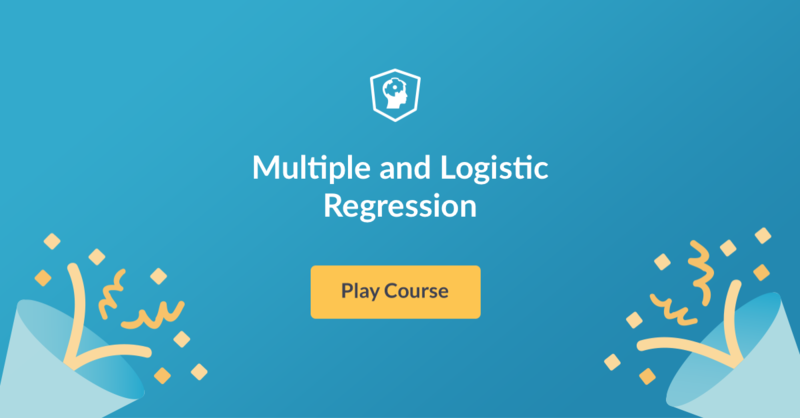# New Statistics Course: Multiple and Logistic Regression!

In this statistics course, you'll learn more about multiple and logistic regression techniques and how to fit, predict, visualize and interpret these models.

Hi there! We just launched a new course: Multiple and Logistic Regression by Ben Baumer!

In this course, you'll take your skills with simple linear regression to the next level. By learning multiple and logistic regression techniques, you will gain the skills to model and predict both numeric and categorical outcomes using multiple input variables. You'll also learn how to fit, visualize, and interpret these models. Then you'll apply your skills to learn about Italian restaurants in New York City!

Take me to chapter 1!

Multiple and Logistic Regression features interactive exercises that combine high-quality video, in-browser coding, and gamification for an engaging learning experience that will make you an expert in regressions!What you'll learn:

Chapter 1: Parallel Slopes

In this chapter, you'll learn about the class of linear models called "parallel slopes models." These include one numeric and one categorical explanatory variable.

Chapter 2: Evacuating and Extending Parallel Slopes Model

This chapter covers model evaluation. By looking at different properties of the model, including the adjusted R-squared, you'll learn to compare models so that you can select the best one. You'll also learn about interaction terms in linear models.

Chapter 3: Multiple Regression

This chapter will show you how to add two, three, and even more numeric explanatory variables to a linear model.

Chapter 4: Logistic Regression

In this chapter you'll learn about using logistic regression, a generalized linear model (GLM), to predict a binary outcome and classify observations.

Chapter 5: Case Study - Italian Restaurants in NYC

Explore the relationship between price and the quality of food, service, and decor for Italian restaurants in NYC.

Learn how to model & predict numeric & categorical outcomes in Multiple & Logistic Regression!